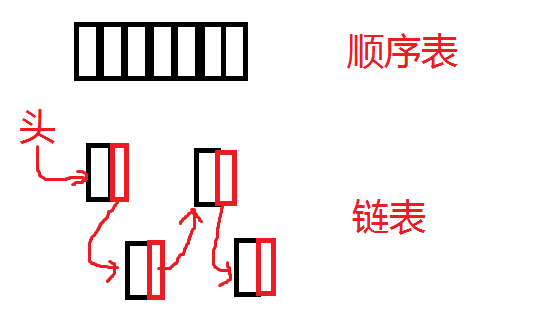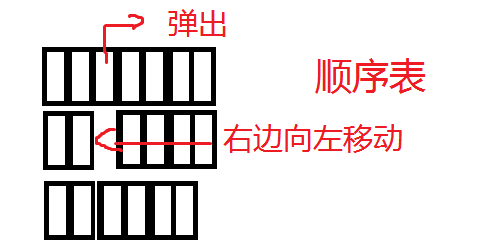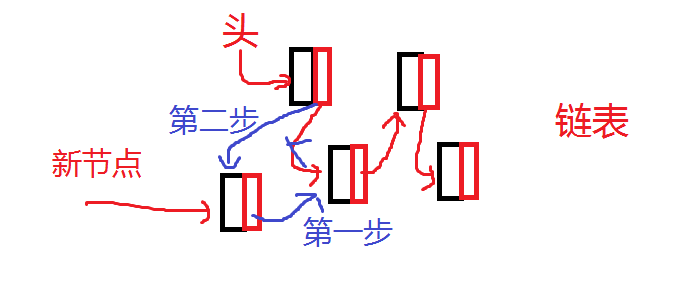# Pyhon3的链表的实现（包含遍历，读取，删除，头插，尾插）## 链表：

class SingleNode(object):
"""单链表的节点"""
def __init__(self, elem):
self.elem = elem  # 存放数据元素
self.next = Nonedef add(self, item):
"""在头部添加元素, 头插法"""
node = SingleNode(item)
self.__length += 1

    def append(self, item):
"""在尾部添加元素,尾插法"""
node = SingleNode(item)
if self.is_empty():
else:
while cur.next != None:
cur = cur.next
cur.next = node
self.__length += 1

def travel(self):
"""遍历整个链表"""
while cur != None:
print(cur.elem, end=' ')
cur = cur.next
print()

class SingleNode(object):
"""单链表的节点"""
def __init__(self, elem):
self.elem = elem  # 存放数据元素
self.next = None

"""单链表的类"""
def __init__(self, node=None):
self.__length = 0
def is_empty(self):  # 因为是对对象的一个操作，所以使用对象的self
"""链表是否为空"""
def length(self):
"""链表长度"""
return self.__length
def travel(self):
"""遍历整个链表"""
while cur != None:
print(cur.elem, end=' ')
cur = cur.next
print()
"""一次读一个"""
while cur != None:
yield cur.elem
cur = cur.next
yield None
"""在头部添加元素, 头插法"""
node = SingleNode(item)
self.__length += 1
def insert(self, pos, item):
"""在指定的位置添加节点,:param pos 从0开始索引"""
if pos <= 0:  # 传进一个不合法的值，为最头部
elif pos > (self.__length-1):
self.append(item)
else:
count = 0
while count < (pos-1):
count += 1
pre = pre.next  # 当循环结束后，pre指向pos-1的位置
node = SingleNode(item)  # 创建一个节点
node.next = pre.next
pre.next = node  # 让pre指向这个节点
self.__length += 1
def append(self, item):
"""在尾部添加元素,尾插法"""
node = SingleNode(item)
if self.is_empty():
else:
while cur.next != None:
cur = cur.next
cur.next = node
self.__length += 1
def remove(self, item):
"""删除节点"""
pre = None
while cur != None:
if cur.elem == item:
if pre == None:  # 当pre为空时，说明还没开始即删除的首节点
self.__length -= 1
return  # 删除之后退出
pre.next = cur.next
cur.next = None
self.__length -= 1
return  # 删除之后退出
pre = cur
cur = cur.next
def search(self, item):
"""查找节点是否存在"""
while cur != None:
if cur.elem == item:
return True
cur = cur.next
return False

def main():
print(ll.is_empty())
print(ll.length())

ll.append(1)
print(ll.is_empty())
print(ll.length())

ll.append(2)
ll.append(3)
ll.travel()
ll.length()
ll.insert(-1, 9)
ll.travel()
ll.insert(2, 90)
ll.travel()
ll.insert(20, 900)
ll.travel()
print(ll.length())
ll.remove(900)
ll.travel()
print(ll.length())
print(ll.search(90))
num1 = next(MyList1_num)
print(num1)

if __name__ == "__main__":
main()


08-07897
04-27346208-29716
10-2187
10-07267
10-12998
07-23207
01-30229
07-203957
01-17154